## Tuesday, November 1, 2016

### Cospeciation method in phytools

Today I have been working on a method to test the hypothesis that two trees are more topologically congruent than expected by chance. This is supposed to be a test for co-speciation.

This test has been used in the literature on a number of occasions (e.g., Logdon et al. 2011); however I'm unsure of the original reference as it doesn't seem to be an article by Rod Page, who has devised a number of methods to study cospeciation.

The method works by first calculating the distance between two input trees by a user specified criterion (e.g., Robinson-Foulds or SPR), then we simulate 100 or more stochastic, uncorrelated trees with labels matching our original phylogenies, finally the function computes a p-value for the test by comparison to this distribution. Note that I also have written `plot` and `print` methods for the function.

Here's an example. First, the functions:

``````cospeciation<-function(t1,t2,distance=c("RF","SPR"),
method=c("simulation","permutation"),assoc=NULL,
nsim=100,...){
distance<-distance
if(!distance%in%c("RF","SPR")) distance<-"RF"
method<-method
if(!method%in%c("simulation","permutation"))
method<-"simulation"
if(is.null(assoc)){
## assume exact match
tips<-intersect(t1\$tip.label,t2\$tip.label)
assoc<-cbind(tips,tips)
}
if(any(!t1\$tip.label%in%assoc[,1]))
t1<-drop.tip(t1,setdiff(t1\$tip.label,assoc[,1]))
if(any(!assoc[,1]%in%t1\$tip.label))
assoc<-assoc[assoc[,1]%in%t1\$tip.label,]
if(any(!t2\$tip.label%in%assoc[,2]))
t2<-drop.tip(t2,setdiff(t2\$tip.label,assoc[,2]))
if(any(!assoc[,2]%in%t2\$tip.label))
assoc<-assoc[assoc[,2]%in%t2\$tip.label,]
if(method=="permutation"){
perm.labels<-function(tree){
tree\$tip.label<-sample(tree\$tip.label)
tree
}
tt1<-replicate(nsim,perm.labels(t1),simplify=FALSE)
swap.t2<-t2
swap.t2\$tip.label<-sapply(t2\$tip.label,function(x,y)
y[which(y[,2]==x),1],y=assoc)
tt2<-replicate(nsim,perm.labels(swap.t2),simplify=FALSE)
} else {
tt1<-pbtree(n=Ntip(t1),tip.label=t1\$tip.label,
nsim=nsim)
swap.t2<-t2
swap.t2\$tip.label<-sapply(t2\$tip.label,function(x,y)
y[which(y[,2]==x),1],y=assoc)
tt2<-pbtree(n=Ntip(t2),tip.label=swap.t2\$tip.label,
nsim=nsim)

}
if(distance=="SPR"){
d.null<-mapply(SPR.dist,tt1,tt2)
d<-SPR.dist(t1,swap.t2)
P.val<-mean(c(d,d.null)<=d)
} else {
d.null<-mapply(RF.dist,tt1,tt2)
d<-RF.dist(t1,swap.t2)
P.val<-mean(c(d,d.null)<=d)
}
obj<-list(d=d,d.null=d.null,P.val=P.val,
distance=if(distance=="SPR") "SPR" else "RF",
method=if(method=="simulation") "simulation" else
"permutation")
class(obj)<-"cospeciation"
obj
}

print.cospeciation<-function(x,...){
cat(paste("\nCo-speciation test based on",x\$distance,
"distance.\n"))
cat(paste("P-value obtained via",x\$method,".\n\n"))
if(x\$distance=="SPR")
cat(paste("   SPR distance:",x\$d,"\n"))
else
cat(paste("   RF distance:",x\$d,"\n"))
cat(paste("   Mean(SD) from null: ",
round(mean(x\$d.null),1),"(",
round(sd(x\$d.null),1),")\n",sep=""))
cat(paste("   P-value:",round(x\$P.val,6),"\n\n"))
}

plot.cospeciation<-function(x,...){
if(x\$distance=="RF")
p<-hist(x\$d.null,breaks=seq(min(c(x\$d,x\$d.null))-3,
max(c(x\$d,x\$d.null))+3,2),plot=FALSE)
else if(x\$distance=="SPR")
p<-hist(x\$d.null,breaks=seq(min(c(x\$d,x\$d.null))-1.5,
max(c(x\$d,x\$d.null))+1.5,1),plot=FALSE)
plot(p\$mids,p\$density,xlim=c(min(c(x\$d,x\$d.null))-2,
max(c(x\$d,x\$d.null))+1),ylim=c(0,1.2*max(p\$density)),
type="n",
xlab=paste(x\$distance," distance (null by ",
x\$method,")",sep=""),ylab="relative frequency")
y2<-rep(p\$density,each=2)
y2<-y2[-length(y2)]
x2<-rep(p\$breaks[2:length(p\$breaks)-1],each=2)[-1]
x3<-c(min(x2),x2,max(x2))
y3<-c(0,y2,0)
polygon(x3,y3,col=make.transparent("blue",0.3),
border=FALSE)
lines(p\$breaks[2:length(p\$breaks)-1],p\$density,type="s")
arrows(x\$d,max(c(0.2*max(p\$density),
1.1*p\$density[which(p\$mids==x\$d)])),
x\$d,0,lend="round",
length=0.15,lwd=2,col="black")
text(x\$d-diff(par()\$usr[1:2])/40,
1.1*max(c(0.2*max(p\$density),
1.1*p\$density[which(p\$mids==x\$d)])),
"observed distance",
srt=60,pos=4)
}
``````

Now our trees. `t1` & `t2` were simulated with co-speciation and host-switching (via SPRs); whereas `t3` is uncorrelated. Finally `a1` maps the tips of `t1` & `t2`; whereas `t1` & `t3` are related via `a2`.

``````library(phytools)
library(phangorn)
t1
``````
``````##
## Phylogenetic tree with 26 tips and 25 internal nodes.
##
## Tip labels:
##  R, F, Q, G, A, P, ...
##
## Rooted; includes branch lengths.
``````
``````t2
``````
``````##
## Phylogenetic tree with 26 tips and 25 internal nodes.
##
## Tip labels:
##  t.R, t.F, t.Q, t.G, t.A, t.P, ...
##
## Rooted; includes branch lengths.
``````
``````a1
``````
``````##       tips tips
##  [1,] "R"  "t.R"
##  [2,] "F"  "t.F"
##  [3,] "Q"  "t.Q"
##  [4,] "G"  "t.G"
##  [5,] "A"  "t.A"
##  [6,] "P"  "t.P"
##  [7,] "X"  "t.X"
##  [8,] "D"  "t.D"
##  [9,] "U"  "t.U"
## [10,] "M"  "t.M"
## [11,] "J"  "t.J"
## [12,] "H"  "t.H"
## [13,] "C"  "t.C"
## [14,] "N"  "t.N"
## [15,] "V"  "t.V"
## [16,] "E"  "t.E"
## [17,] "O"  "t.O"
## [18,] "S"  "t.S"
## [19,] "T"  "t.T"
## [20,] "Y"  "t.Y"
## [21,] "Z"  "t.Z"
## [22,] "I"  "t.I"
## [23,] "K"  "t.K"
## [24,] "W"  "t.W"
## [25,] "B"  "t.B"
## [26,] "L"  "t.L"
``````
``````plot(cophylo(t1,t2,assoc=a1))
``````
``````## Rotating nodes to optimize matching...
## Done.
``````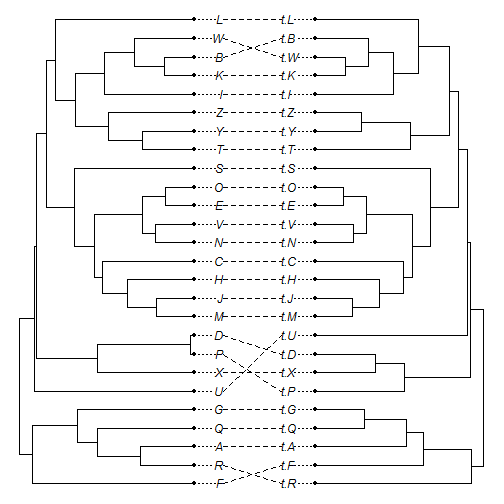``````co1<-cospeciation(t1,t2,assoc=a1)
co1
``````
``````##
## Co-speciation test based on RF distance.
## P-value obtained via simulation .
##
##    RF distance: 16
##    Mean(SD) from null: 36.6(4.6)
##    P-value: 0.009901
``````
``````plot(co1)
``````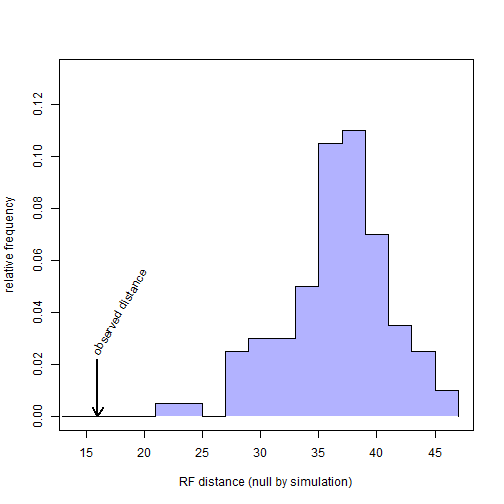``````t3
``````
``````##
## Phylogenetic tree with 26 tips and 25 internal nodes.
##
## Tip labels:
##  r, w, o, g, n, t, ...
##
## Rooted; includes branch lengths.
``````
``````a2
``````
``````##       LETTERS letters
##  [1,] "A"     "a"
##  [2,] "B"     "b"
##  [3,] "C"     "c"
##  [4,] "D"     "d"
##  [5,] "E"     "e"
##  [6,] "F"     "f"
##  [7,] "G"     "g"
##  [8,] "H"     "h"
##  [9,] "I"     "i"
## [10,] "J"     "j"
## [11,] "K"     "k"
## [12,] "L"     "l"
## [13,] "M"     "m"
## [14,] "N"     "n"
## [15,] "O"     "o"
## [16,] "P"     "p"
## [17,] "Q"     "q"
## [18,] "R"     "r"
## [19,] "S"     "s"
## [20,] "T"     "t"
## [21,] "U"     "u"
## [22,] "V"     "v"
## [23,] "W"     "w"
## [24,] "X"     "x"
## [25,] "Y"     "y"
## [26,] "Z"     "z"
``````
``````plot(cophylo(t1,t3,assoc=a2))
``````
``````## Rotating nodes to optimize matching...
## Done.
``````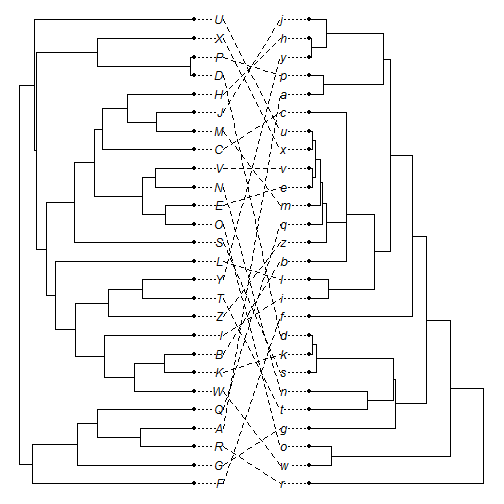``````co2<-cospeciation(t1,t3,assoc=a2)
co2
``````
``````##
## Co-speciation test based on RF distance.
## P-value obtained via simulation .
##
##    RF distance: 46
##    Mean(SD) from null: 45.2(1.1)
##    P-value: 1
``````
``````plot(co2)
``````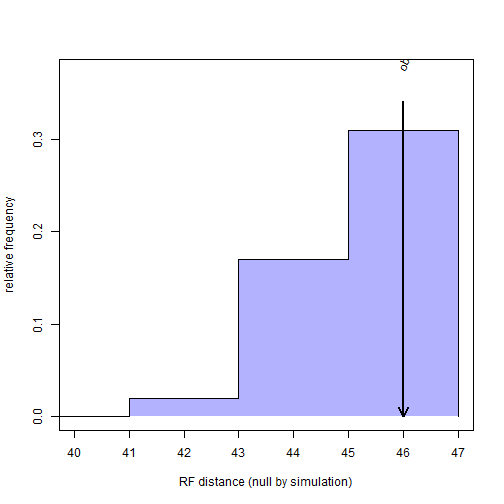OK, well, the `plot` method may still need some work, but you get the idea.

#### 1 comment:

1.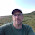That's cool. Package distory provides a geodesic distance that also be used to this end. The test, in any case, is restricted to one-to-one associations (that is, trees of the same size). I am working on a way to apply a similar approach to multiple "host-parasite" associations (or trees of different sizes).

Note: due to the very large amount of spam, all comments are now automatically submitted for moderation.